Ex 10.3

Chapter 10 Class 6 Mensuration
Serial order wise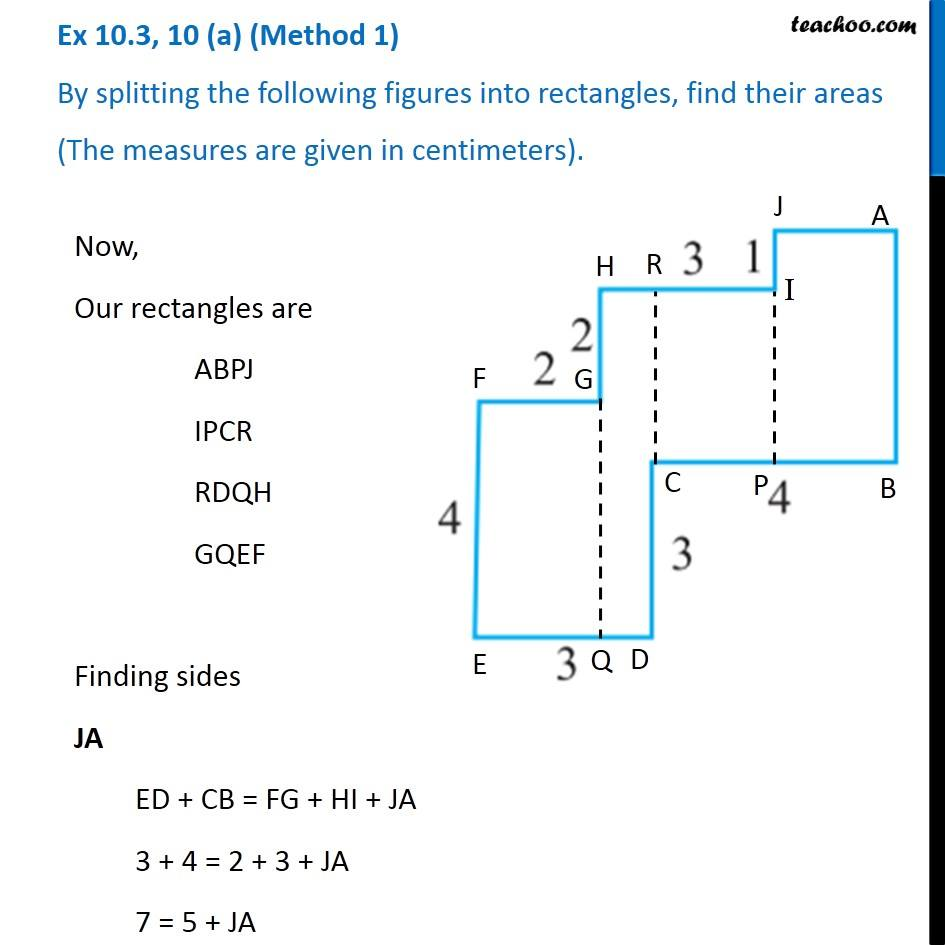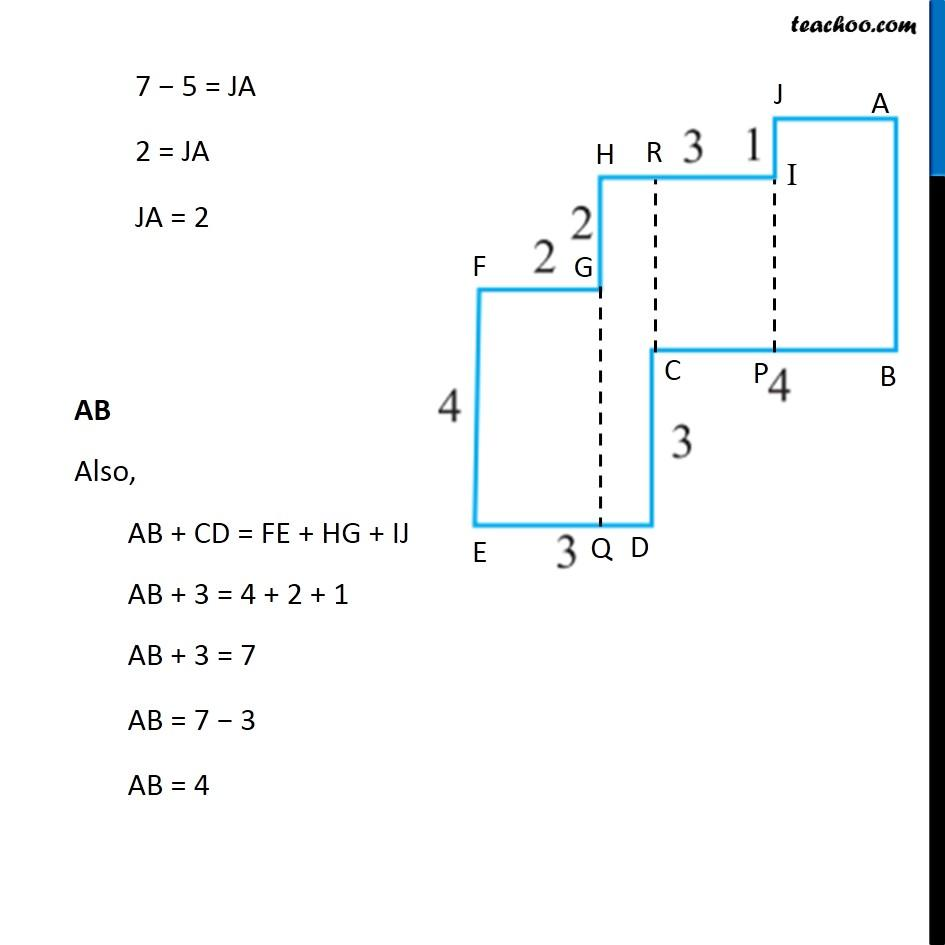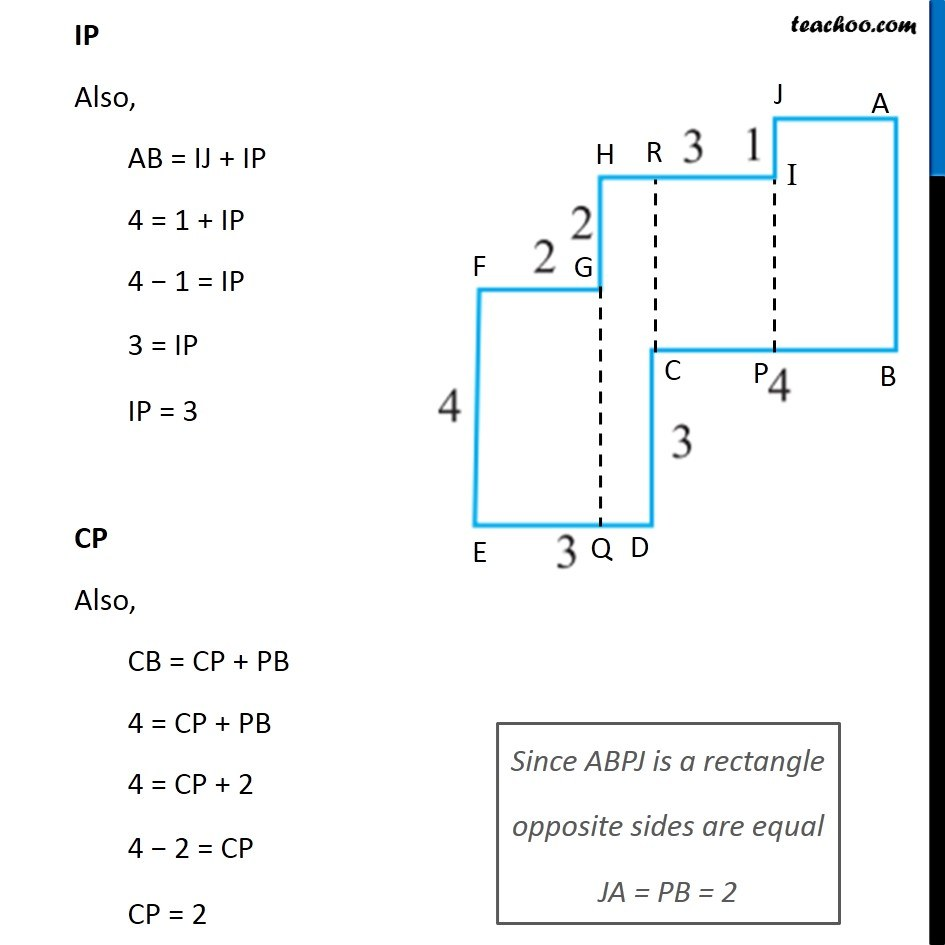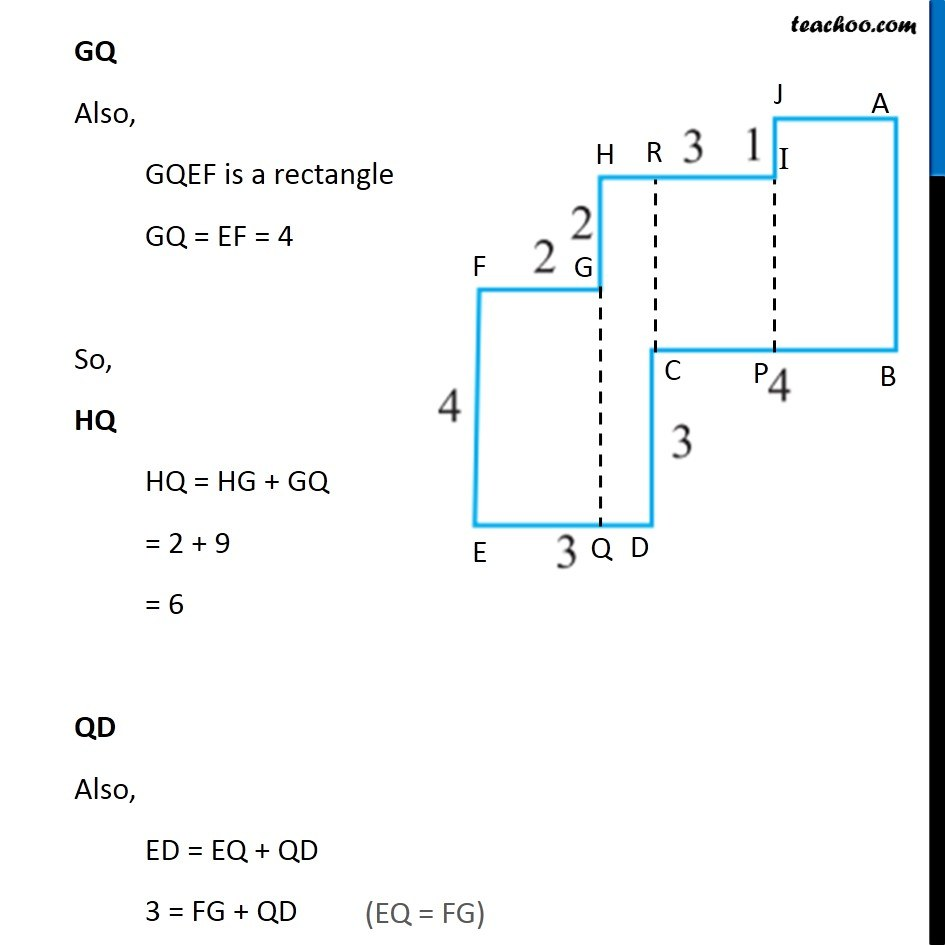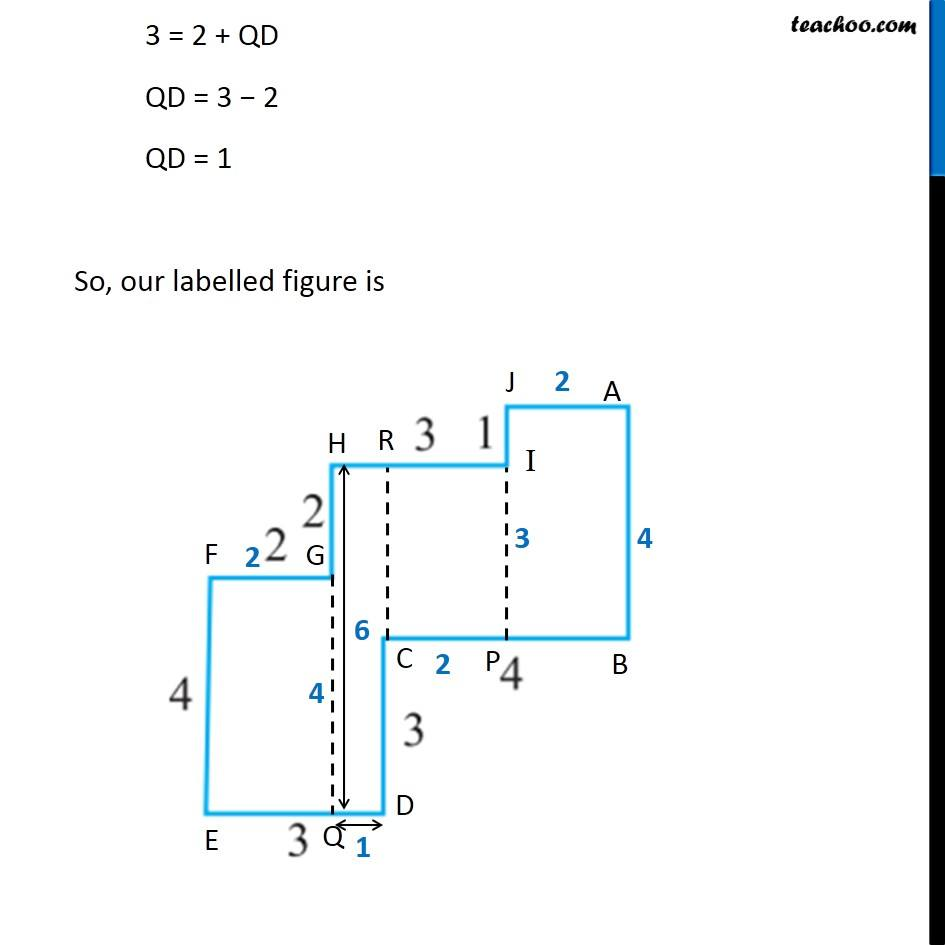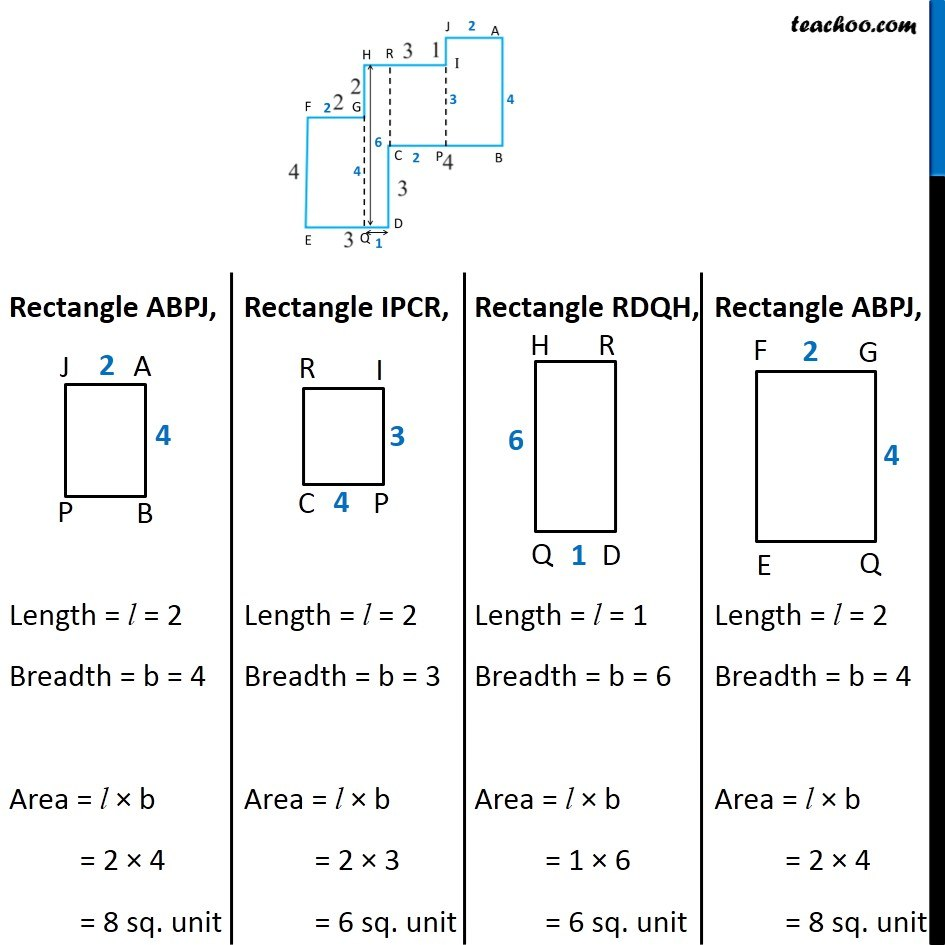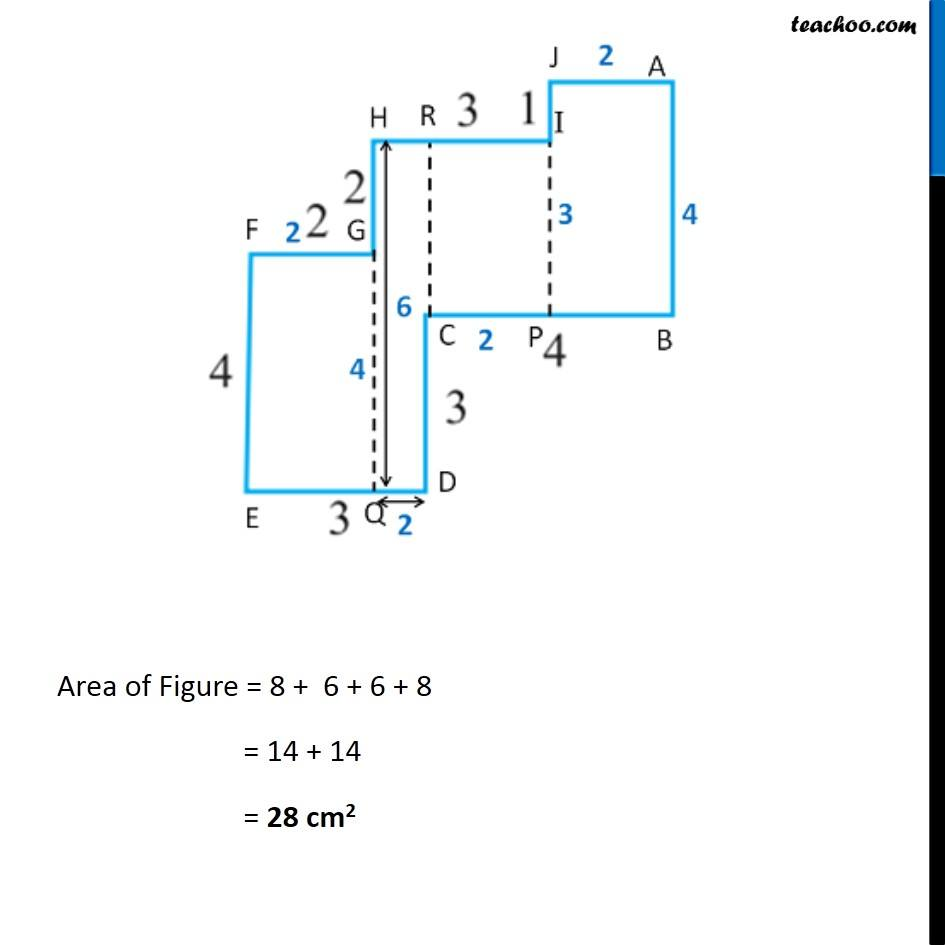Get live Maths 1-on-1 Classs - Class 6 to 12

### Transcript

Ex 10.3, 10 (a) (Method 1) By splitting the following figures into rectangles, find their areas (The measures are given in centimeters). Now, Our rectangles are ABPJ IPCR RDQH GQEF Finding sides JA ED + CB = FG + HI + JA 3 + 4 = 2 + 3 + JA 7 = 5 + JA 7 − 5 = JA 2 = JA JA = 2 AB Also, AB + CD = FE + HG + IJ AB + 3 = 4 + 2 + 1 AB + 3 = 7 AB = 7 − 3 AB = 4 IP Also, AB = IJ + IP 4 = 1 + IP 4 − 1 = IP 3 = IP IP = 3 CP Also, CB = CP + PB 4 = CP + PB 4 = CP + 2 4 − 2 = CP CP = 2 Since ABPJ is a rectangle opposite sides are equal JA = PB = 2 GQ Also, GQEF is a rectangle GQ = EF = 4 So, HQ HQ = HG + GQ = 2 + 9 = 6 QD Also, ED = EQ + QD 3 = FG + QD (EQ = FG) 3 = 2 + QD QD = 3 − 2 QD = 1 So, our labelled figure is Rectangle ABPJ, Length = l = 2 Breadth = b = 4 Area = l × b = 2 × 4 = 8 sq. unit Rectangle IPCR, Length = l = 2 Breadth = b = 3 Area = l × b = 2 × 3 = 6 sq. unit Rectangle RDQH, Length = l = 1 Breadth = b = 6 Area = l × b = 1 × 6 = 6 sq. unit Rectangle ABPJ, Length = l = 2 Breadth = b = 4 Area = l × b = 2 × 4 = 8 sq. unit Area of Figure = 8 + 6 + 6 + 8 = 14 + 14 = 28 cm2 Ex 10.3, 10 (a) (Method 2) By splitting the following figures into rectangles, find their areas (The measures are given in centimeters). Now, Our rectangles are APIJ PQGH QBRF CDER Finding sides, BR BR = ED + BC BR = 3 + 4 BR = 7 FR EF = ER + FR 4 = CD + FR 4 = 3 + FR FR = 4 − 3 FR = 1 GQ ED + CB = FG + GQ 3 + 4 = 2 + GQ 7 = 2 + GQ 7 − 2 = GQ 5 = GQ GQ = 5 In rectangle CDER, Oposite sides are equal ∴ CD = ER) JA ED + CB = FG + HI + JA 3 + 4 = 2 + 3 + JA 7 = 5 + JA 7 − 5 = JA JA = 2 So, our labeled figure is, Rectangle APIJ, Length = l = 2 Breadth = b = 1 Area = l × b = 2 × 1 = 2 cm2 Rectangle PQGH, Length = l = 5 Breadth = b = 2 Area = l × b = 5 × 2 = 10 cm2 Rectangle QBRF, Length = l = 7 Breadth = b = 1 Area = l × b = 7 × 1 = 7 cm2 Rectangle CDER, Length = l = 3 Breadth = b = 3 Area = l × b = 3 × 3 = 9 cm2 Area of Figure = 2 + 10 + 7 + 9 = 12 + 16 = 28 cm2